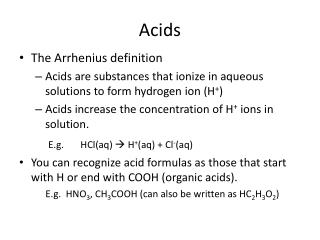DownloadDownload PresentationAcids

# Acids

Download Presentation## Acids

- - - - - - - - - - - - - - - - - - - - - - - - - - - E N D - - - - - - - - - - - - - - - - - - - - - - - - - - -
##### Presentation Transcript

1. Acids • The Arrhenius definition • Acids are substances that ionize in aqueous solutions to form hydrogen ion (H+) • Acids increase the concentration of H+ ions in solution. E.g. HCl(aq)  H+(aq) + Cl-(aq) • You can recognize acid formulas as those that start with H or end with COOH (organic acids). E.g. HNO3, CH3COOH (can also be written as HC2H3O2)

2. Acids • Strong Acids: Completely dissociate into anion and H+ (strong electrolytes) E.g. HCl(aq)  H+(aq) + Cl-(aq) • List of Seven strong acids: • HCl, HBr, HI, HNO3, H2SO4, HClO3, HClO4 • Weak Acids: Ionize slightly in water (weak electrolyte) • Acids other than the seven common strong acids • E.g. HF, HCN • MEMORIZE THE 7 STRONG ACIDS HF(aq) H+(aq) + F-(aq)

3. Basess • The Arrhenius definition • Bases are substances that ionize in aqueous solutions to form hydroxide ion (OH-) • Bases increase the concentration of OH- ions in water. E.g. NaOH(aq)  Na+(aq) + OH-(aq) • You can recognize base formulas as those that end with OH or bases that do not contain OH usually contain H and N or C, H, and N. E.g. Ca(OH)2, NH3, C5H5N

4. Bases • List of Strong bases: • Group 1A metal hydroxides and heavy group IIA metal hydroxides • LiOH, NaOH, KOH, RbOH, CsOH, Ca(OH)2, Ba(OH)2, Sr(OH)2 • Weak bases: Compounds containing N and H or C, H and N • E.g. NH3, C2H5NH2 MEMORIZE THE LIST OF 8 STRONG BASES

5. Salts • A salt is an ionic compound that is neither an acid nor a base. To identify a salt, look for a formula that contains a cation that is not H+ and an anion that is not OH−. • Usually made of a metal + nonmetal • E.g. NaCl, Zn(NO3)2

6. Practice: Identify the following as an acid, a base, or a salt • HNO2 _______ • CsOH _______ • NH4OH _______ • HCN _______ • NH4Br _______ • C3H5NH2 _______ • K2SO4 _______